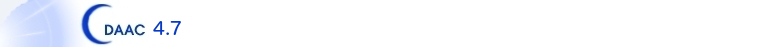Home Page Data Center Research Tools Post Process Results Climate Processing
 Data Access Data Download Interface File Formats Documentation

Name Template: PUB/MISSION/level1a/leoAtt/leoAtt_YYYY.DDD.LLL.NN_txt

 DDD Day of year LLL LEO id MISSION Mission ID NN dump number PUB Base directory for the pub hierarchy YYYY Year

Attitude and rough position data from the COSMIC spacecraft andthe GOX navigation solutions. These are ASCII text files following the format for CHAMP attitude data developed by GFZ with some extensions. Here is the documentation from a leoAtt file header.
```COSMIC attitude data from ATT records and GOX NAV solutions
Attitude mode: -xAft
Data type:
tim:  GPS time: yr, mon, day, hr, min, sec
att:  Inertial attitude, as expected by Bernese:
0000 qx, qy, qz, qw 0.00.  Quaternion to convert from Inertial True-of-Epoch (ITOE)
frame to spacecraft frame (described below under sca as the Bernese local level frame).
That is, a vector, v, in the
ITOE frame has coordinates v_prime = q*vq (* is conjugated) in the spacecraft frame.
The opposite transform: A vector, v, in the spacecraft frame, has coordinates
v_prime = qvq* in the ITOE frame.
sca:  Quaternion to convert Spacecraft reference frame:
Z nadir (TIP) direction,
X antenna direction (+X antenna, nominal anti-velocity direction)
Y perpendicular to disk of satellite, to the left as one views the -X direction
as one stands on top of the satellite
to orbit local level frame:
Z = nadir = -r/|r|      (where r is s/c position vector)
Y = (r x v) / | r x v | (where v is s/c velocity vector)
X = Y x Z               (where x is vector cross product)
r and v are the position and velocity in the ITOE frame.
NOTE:  This is not the local level frame Bernese uses, but the frame defined
by Orbital sciences.  These are the quaternions straight from the spacecraft
in the Orbital local level frame.

The Orbital local level frame is:
+Y to left as you stand on top of s/c facing toward velocity direction
----X  +Z into page, to nadir
|
|
V  +X (nominal anti-velocity)

But the Bernese local level frame (used in computing the 'att' quaternion above) is:
+X (nominal velocity direction)
|
|
X----> +Y
+Z into page, to nadir
A vector, u, in the spacecraft frame, has coordinates uprime = q*uq in the local
level frame.
All quaterions (type att and sca) are given in order: qx, qy, qz, qw
where qw = cos(theta/2) and q(xyz) = ehat * sin(theta/2)
sad:  Solar array drive angle (deg)
rat:  Attitude rates:  rollRate, pitchRate, yawRate (deg/sec)
ang:  Euler angles:  Bank (roll) angle (deg):       Angle about x-axis
Elevation (pitch) angle (deg): Angle about y-axis
Heading (yaw) angle (deg):     Angle about z-axis
If the yaw angle is close to zero, this means the
S/C is in normal yaw mode (+X antenna aft)
All angles are in the right-hand sense and follows the so-called Aerospace sequence:
1) Heading (yaw): the angle about +Z local level axis counted positive from +X toward +Y
2) Elevation (pitch): the angle about new y-axis counted positive from new +z toward new +x
3) Bank (roll): the angle about new x-axis counted positive from new +y toward new +z
pve:  Position and velocity in Earth Centered Fixed coordinates (km)
X, Y, Z, Xdot, Ydot, Zdot
pvi:  Position and velocity in Inertial True-of-Epoch coordinates (km)
X, Y, Z, Xdot, Ydot, Zdot
```